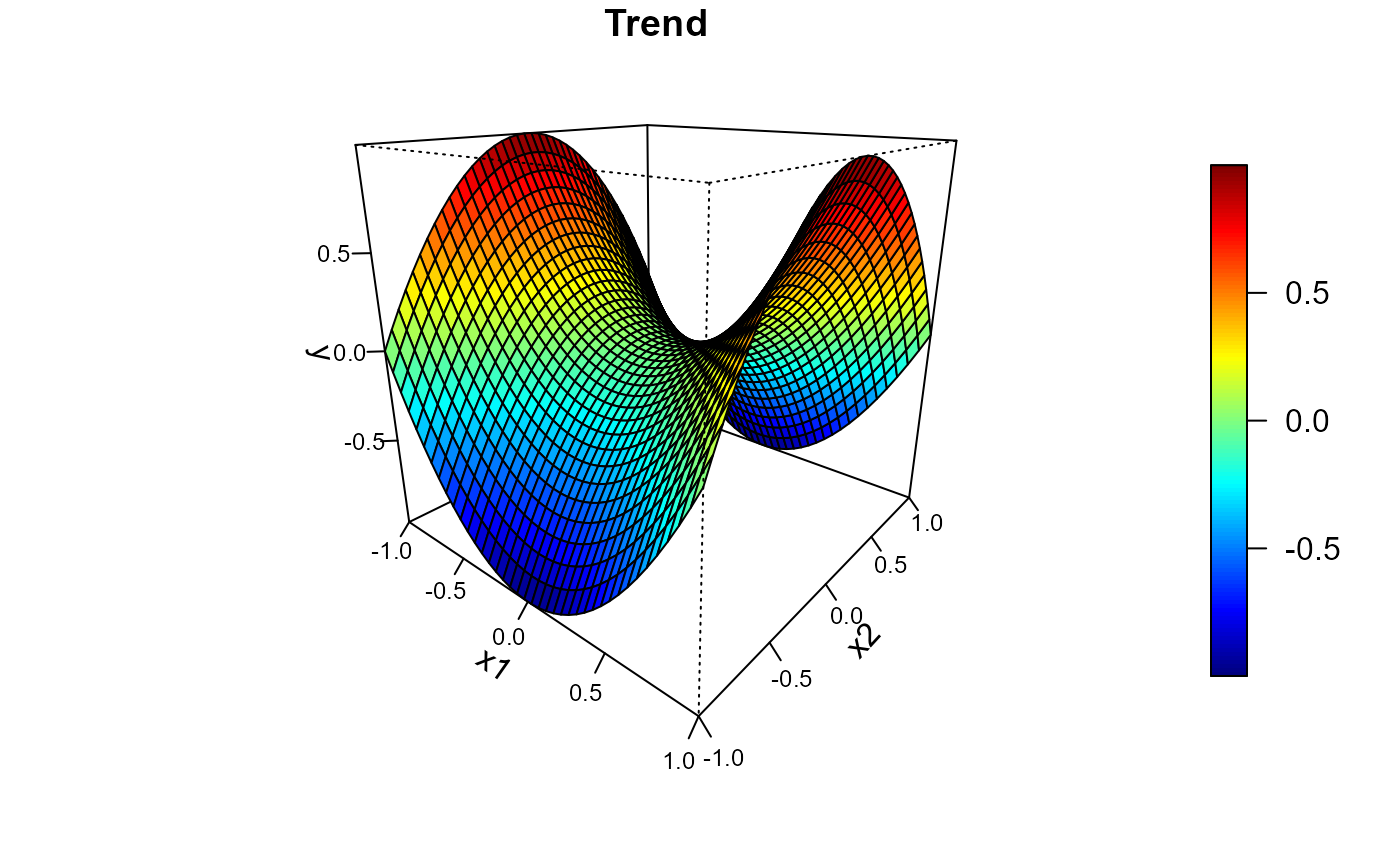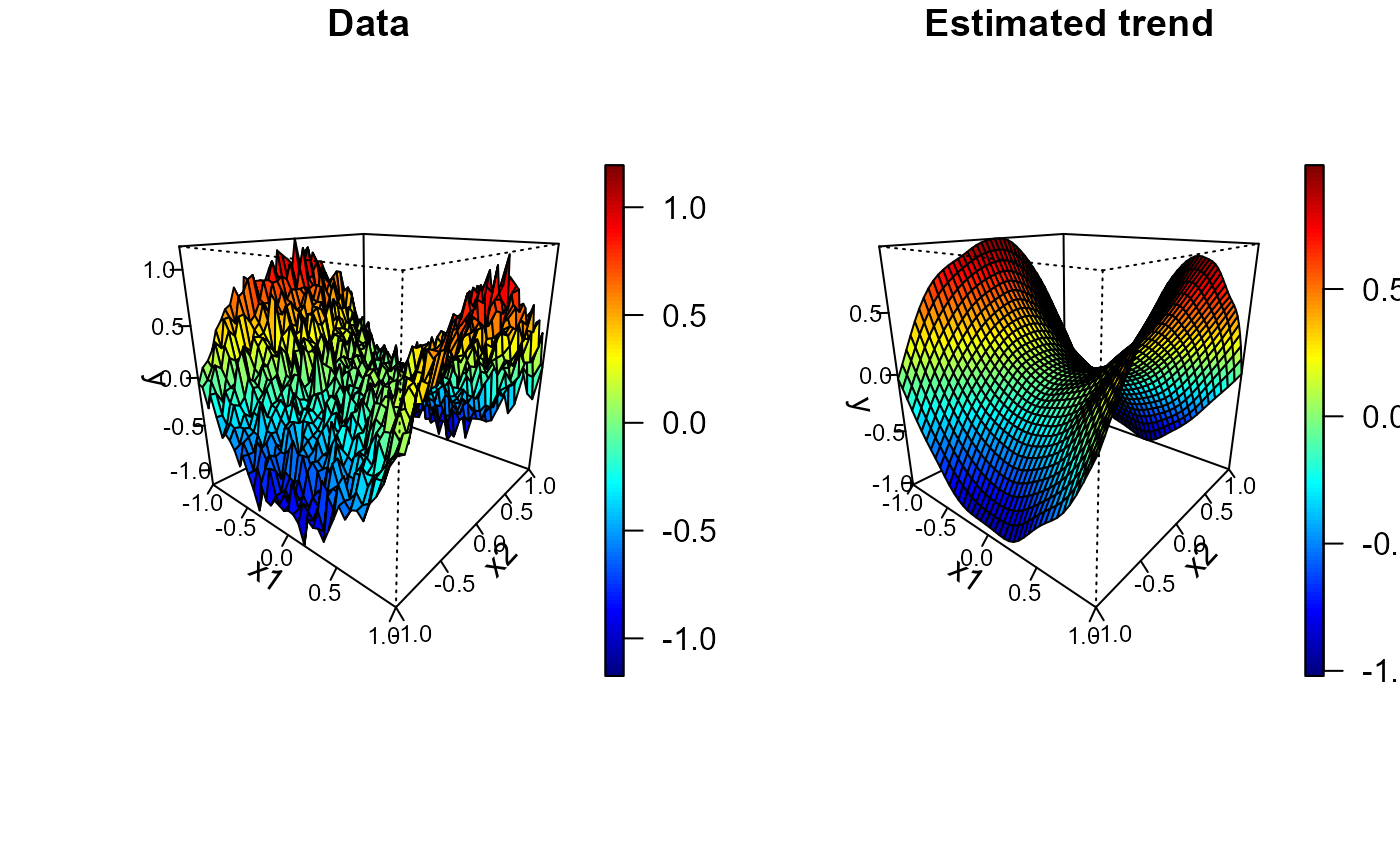spersp (generic function) draws a perspective plot of a surface over the x-y plane with the facets being filled with different colors and (optionally) adds a legend strip with the color scale (calls splot and persp).

spersp(x, ...)

# S3 method for default
spersp(
x = seq(0, 1, len = nrow(z)),
y = seq(0, 1, len = ncol(z)),
z,
s = z,
slim = range(s, finite = TRUE),
col = jet.colors(128),
breaks = NULL,
legend = TRUE,
horizontal = FALSE,
legend.shrink = 0.8,
legend.width = 1.2,
legend.mar = ifelse(horizontal, 3.1, 5.1),
legend.lab = NULL,
bigplot = NULL,
smallplot = NULL,
lab.breaks = NULL,
axis.args = NULL,
legend.args = NULL,
graphics.reset = FALSE,
xlab = NULL,
ylab = NULL,
zlab = NULL,
theta = 40,
phi = 20,
ticktype = "detailed",
cex.axis = 0.75,
...
)

# S3 method for data.grid
spersp(
x,
data.ind = 1,
s = x[[data.ind]],
xlab = NULL,
ylab = NULL,
zlab = NULL,
...
)

## Arguments

x grid values for x coordinate. If x is a list, its components x$x and x$y are used for x and y, respectively. If the list has component z this is used for z. additional graphical parameters (to be passed to persp or spersp.default; e.g. xlim, ylim, zlim, ...). NOTE: graphical arguments passed here will only have impact on the main plot. To change the graphical defaults for the legend use the par function beforehand (e.g. par(cex.lab = 2) to increase colorbar labels). grid values for y coordinate. matrix containing the values to be plotted (NAs are allowed). Note that x can be used instead of z for convenience. matrix containing the values used for coloring the facets. limits used to set up the color scale. color table used to set up the color scale (see image for details). (optional) numeric vector with the breakpoints for the color scale: must have one more breakpoint than col and be in increasing order. logical; if TRUE (default), the plotting region is splitted into two parts, drawing the perspective plot in one and the legend with the color scale in the other. If FALSE only the (coloured) perspective plot is drawn and the arguments related to the legend are ignored (splot is not called). logical; if FALSE (default) legend will be a vertical strip on the right side. If TRUE the legend strip will be along the bottom. amount to shrink the size of legend relative to the full height or width of the plot. width in characters of the legend strip. Default is 1.2, a little bigger that the width of a character. width in characters of legend margin that has the axis. Default is 5.1 for a vertical legend and 3.1 for a horizontal legend. label for the axis of the color legend. Default is no label as this is usual evident from the plot title. plot coordinates for main plot. If not passed these will be determined within the function. plot coordinates for legend strip. If not passed these will be determined within the function. if breaks are supplied these are text string labels to put at each break value. This is intended to label axis on a transformed scale such as logs. additional arguments for the axis function used to create the legend axis (see image.plot for details). arguments for a complete specification of the legend label. This is in the form of list and is just passed to the mtext function. Usually this will not be needed (see image.plot for details). logical; if FALSE (default) the plotting region (par("plt")) will not be reset to make it possible to add more features to the plot (e.g. using functions such as points or lines). If TRUE will reset plot parameters to the values before entering the function. label for the x axis, defaults to a description of x. label for the y axis, defaults to a description of y. label for the z axis, defaults to a description of z. x-y rotation angle for perspective (azimuthal direction). z-angle for perspective (colatitude). character; "simple" draws just an arrow parallel to the axis to indicate direction of increase; "detailed" draws normal ticks as per 2D plots. magnification to be used for axis annotation (relative to the current setting of par("cex")). integer (or character) with the index (or name) of the component containing the z values to be plotted.

## Value

Invisibly returns a list with the following 4 components:

pm

the viewing transformation matrix (see persp for details), a 4 x 4 matrix that can be used to superimpose additional graphical elements using the function trans3d.

bigplot

plot coordinates of the main plot. These values may be useful for drawing a plot without the legend that is the same size as the plots with legends.

smallplot

plot coordinates of the secondary plot (legend strip).

old.par

previous graphical parameters (par(old.par) will reset plot parameters to the values before entering the function).

## Side Effects

After exiting, the plotting region may be changed (par("plt")) to make it possible to add more features to the plot (set graphics.reset = FALSE to avoid this).

splot, spoints, simage, image, image.plot, data.grid, persp.

## Author

Based on image.plot function from package fields: fields, Tools for spatial data. Copyright 2004-2013, Institute for Mathematics Applied Geosciences. University Corporation for Atmospheric Research.

Modified by Ruben Fernandez-Casal <rubenfcasal@gmail.com>.

## Examples


#
# Regularly spaced 2D data
nx <- c(40, 40) # ndata =  prod(nx)
x1 <- seq(-1, 1, length.out = nx)
x2 <- seq(-1, 1, length.out = nx)
trend <- outer(x1, x2, function(x,y) x^2 - y^2)
spersp( x1, x2, trend, main = 'Trend', zlab = 'y')#
# Multiple plots
set.seed(1)
y <- trend + rnorm(prod(nx), 0, 0.1)
x <- as.matrix(expand.grid(x1 = x1, x2 = x2)) # two-dimensional grid
# local polynomial kernel regression
lp <- locpol(x, y, nbin = nx, h =  diag(c(0.3, 0.3)))
# 1x2 plot
old.par <- par(mfrow = c(1,2))
spersp( x1, x2, y, main = 'Data')
spersp(lp, main = 'Estimated trend', zlab = 'y')par(old.par)